• 此为本人MATLAB图像处理课的大作业，对于初学者可以参考Matlab数字图像处理
• 数字图像处理MATLAB实现（第2版）每章配套图片和代码，主编杨杰
• 显示索引图像和灰度图像&gt;&gt; [X,map]=imread('trees.tif');&gt;&gt; gmap=rgb2gray(map);&gt;&gt; figure,imshow(X,map);&gt;&gt; figure,imshow(X,gmap);利用膨胀函数平移图像...
显示索引图像和灰度图像>> [X,map]=imread('trees.tif');>> gmap=rgb2gray(map);>> figure,imshow(X,map);>> figure,imshow(X,gmap);利用膨胀函数平移图像I = imread('football.jpg');se = translate(strel(1), [30 30]);%将一个平面结构化元素分别向下和向右移动30个位置J = imdilate(I,se);%利用膨胀函数平移图像subplot(121);imshow(I), title('原图')subplot(122), imshow(J), title('移动后的图像');水平翻转和上下翻转I = imread('cameraman.tif');Flip1=fliplr(I);               %  对矩阵I左右反转subplot(131);imshow(I);title('原图');subplot(132);imshow(Flip1);title('水平镜像');Flip2=flipud(I);               %  对矩阵I垂直反转subplot(133);imshow(Flip2);title('竖直镜像');图像旋转I=imread('cameraman.tif');B=imrotate(I,60,'bilinear','crop');%双线性插值法旋转图像，并裁剪图像，使其和原图像大小一致subplot(121),imshow(I),title('原图');subplot(122),imshow(B),title('旋转图像60^{o}，并剪切图像');截取图像I = imread('circuit.tif');I2 = imcrop(I,[75 68 130 112]);imshow(I), figure, imshow(I2)画轮廓>> I=imread('circuit.tif');>> imshow(I)>> figure>> imcontour(I,3)噪声和滤波I=imread('cameraman.tif');J=imnoise(I,'salt & pepper',0.02);%添加椒盐噪声subplot(121),imshow(J);title('噪声图像')K=medfilt2(J);%使用3*3的邻域窗的中值滤波subplot(122),imshow(K);title('中值滤波后图像')Matlab数字数字图像处理函数汇总：1、数字数字图像的变换① fft2：fft2函数用于数字数字图像的二维傅立叶变换，如：i=imread('104_8.tif');j=fft2(i);②ifft2:：ifft2函数用于数字数字图像的二维傅立叶反变换，如： i=imread('104_8.tif'); j=fft2(i);k=ifft2(j);2、模拟噪声生成函数和预定义滤波器① imnoise：用于对数字数字图像生成模拟噪声，如： i=imread('104_8.tif'); j=imnoise(i,'gaussian',0,0.02);%模拟高斯噪声② fspecial：用于产生预定义滤波器，如：h=fspecial('sobel');%sobel水平边缘增强滤波器h=fspecial('gaussian');%高斯低通滤波器h=fspecial('laplacian');%拉普拉斯滤波器h=fspecial('log');%高斯拉普拉斯（LoG）滤波器h=fspecial('average');%均值滤波器2、数字数字图像的增强①直方图：imhist函数用于数字数字图像的直方图显示，如：i=imread('104_8.tif');imhist(i);②直方图均化：histeq函数用于数字数字图像的直方图均化，如：i=imread('104_8.tif');j=histeq(i);imshow(J)③对比度调整：imadjust函数用于数字数字图像的对比度调整，如：i=imread('104_8.tif');j=imadjust(i,[0.3,0.7],[]);④对数变换：log函数用于数字数字图像的对数变换，如：i=imread('104_8.tif');j=double(i);k=log(j);⑤基于卷积的数字数字图像滤波函数：filter2函数用于数字数字图像滤波，如：i=imread('104_8.tif');h=[1,2,1;0,0,0;-1,-2,-1];j=filter2(h,i);⑥线性滤波：利用二维卷积conv2滤波, 如:i=imread('104_8.tif');h=[1,1,1;1,1,1;1,1,1];h=h/9;j=conv2(i,h);⑦中值滤波：medfilt2函数用于数字数字图像的中值滤波，如：i=imread('104_8.tif');j=medfilt2(i);⑧锐化（1）利用Sobel算子锐化数字数字图像, 如:i=imread('104_8.tif');h=[1,2,1;0,0,0;-1,-2,-1];%Sobel算子j=filter2(h,i);（2）利用拉氏算子锐化数字数字图像, 如:i=imread('104_8.tif');j=double(i);h=[0,1,0;1,-4,0;0,1,0];%拉氏算子k=conv2(j,h,'same');m=j-k;3、数字数字图像边缘检测①sobel算子 如：i=imread('104_8.tif');j =edge(i,'sobel',thresh) ②prewitt算子 如：i=imread('104_8.tif');j =edge(i,'prewitt',thresh)③roberts算子  如：i=imread('104_8.tif');j =edge(i,'roberts',thresh)④log算子  如：i=imread('104_8.tif');j =edge(i,'log',thresh)⑤canny算子 如：i=imread('104_8.tif');j =edge(i,'canny',thresh)⑥Zero-Cross算子 如：i=imread('104_8.tif');j =edge(i,'zerocross',thresh)4、形态学数字数字图像处理①膨胀：是在二值化数字数字图像中“加长”或“变粗”的操作，函数imdilate执行膨胀运算，如：a=imread('104_7.tif');%输入二值数字数字图像b=[0 1 0;1 1 1;01 0];c=imdilate(a,b);②腐蚀：函数imerode执行腐蚀，如：a=imread('104_7.tif');%输入二值数字数字图像b=strel('disk',1);c=imerode(a,b);③开运算：先腐蚀后膨胀称为开运算，用imopen来实现，如： a=imread('104_8.tif');b=strel('square',2);c=imopen(a,b);④闭运算：先膨胀后腐蚀称为闭运算，用imclose来实现，如： a=imread('104_8.tif');b=strel('square',2);c=imclose(a,b);  数字数字图像增强1. 直方图均衡化的 Matlab 实现1.1 imhist 函数功能：计算和显示数字数字图像的色彩直方图格式：imhist(I,n)        imhist(X,map)说明：imhist(I,n) 其中，n 为指定的灰度级数目，缺省值为256；imhist(X,map) 就算和显示索引色数字数字图像 X 的直方图，map为调色板。用stem(x,counts) 同样可以显示直方图。1.2 imcontour 函数功能：显示数字数字图像的等灰度值图格式：imcontour(I,n),imcontour(I,v)说明：n 为灰度级的个数，v 是有用户指定所选的等灰度级向量。1.3 imadjust 函数功能：通过直方图变换调整对比度格式：J=imadjust(I,[low high],[bottomtop],gamma)        newmap=imadjust(map,[low high],[bottomtop],gamma)说明：J=imadjust(I,[low high],[bottomtop],gamma) 其中，gamma 为校正量r，[lowhigh] 为原数字数字图像中要变换的灰度范围，[bottom top]指定了变换后的灰度范围；newmap=imadjust(map,[lowhigh],[bottom top],gamma) 调整索引色数字数字图像的调色板 map 。此时若 [low high] 和[bottom top] 都为2×3的矩阵，则分别调整 R、G、B 3个分量。1.4 histeq 函数功能：直方图均衡化格式：J=histeq(I,hgram)        J=histeq(I,n)        [J,T]=histeq(I,...)        newmap=histeq(X,map,hgram)        newmap=histeq(X,map)        [new,T]=histeq(X,...)说明：J=histeq(I,hgram) 实现了所谓“直方图规定化”，即将原是图象 I 的直方图变换成用户指定的向量 hgram 。hgram 中的每一个元素都在 [0,1] 中；J=histeq(I,n) 指定均衡化后的灰度级数 n ，缺省值为 64；[J,T]=histeq(I,...)返回从能将数字数字图像 I 的灰度直方图变换成数字数字图像 J 的直方图的变换 T ；newmap=histeq(X,map) 和 [new,T]=histeq(X,...) 是针对索引色数字数字图像调色板的直方图均衡。2. 噪声及其噪声的 Matlab 实现        imnoise 函数格式：J=imnoise(I,type)        J=imnoise(I,type,parameter)说明：J=imnoise(I,type) 返回对数字数字图像 I 添加典型噪声后的有噪数字数字图像 J ，参数type 和 parameter 用于确定噪声的类型和相应的参数。3. 数字数字图像滤波的 Matlab 实现3.1 conv2 函数功能：计算二维卷积格式：C=conv2(A,B)        C=conv2(Hcol,Hrow,A)        C=conv2(...,'shape')说明：对于 C=conv2(A,B) ，conv2 的算矩阵A 和 B 的卷积，若[Ma,Na]＝size(A), [Mb,Nb]=size(B), 则 size(C)=[Ma+Mb-1,Na+Nb-1];C=conv2(Hcol,Hrow,A) 中，矩阵 A 分别与Hcol 向量在列方向和 Hrow 向量在行方向上进行卷积；C=conv2(...,'shape') 用来指定 conv2返回二维卷积结果部分，参数 shape 可取值如下：        》full为缺省值，返回二维卷积的全部结果；        》same返回二维卷积结果中与 A 大小相同的中间部分；        valid 返回在卷积过程中，未使用边缘补 0 部分进行计算的卷积结果部分，当 size(A)>size(B) 时，size(C)=[Ma-Mb+1,Na-Nb+1]。3.2 conv 函数功能：计算多维卷积格式：与 conv2 函数相同3.3 filter2函数功能：计算二维线型数字滤波，它与函数 fspecial 连用格式：Y=filter2(B,X)        Y=filter2(B,X,'shape')说明：对于 Y=filter2(B,X) ，filter2 使用矩阵B 中的二维 FIR 滤波器对数据 X 进行滤波，结果 Y 是通过二维互相关计算出来的，其大小与 X 一样；对于Y=filter2(B,X,'shape') ，filter2返回的 Y 是通过二维互相关计算出来的，其大小由参数 shape 确定，其取值如下：        》full返回二维相关的全部结果，size(Y)>size(X)；        》same返回二维互相关结果的中间部分，Y 与X 大小相同；        》valid返回在二维互相关过程中，未使用边缘补 0 部分进行计算的结果部分，有 size(Y)<size(X) 。3.4 fspecial 函数功能：产生预定义滤波器格式：H=fspecial(type)        H=fspecial('gaussian',n,sigma)        高斯低通滤波器        H=fspecial('sobel')                        Sobel 水平边缘增强滤波器        H=fspecial('prewitt')                     Prewitt 水平边缘增强滤波器        H=fspecial('laplacian',alpha)            近似二维拉普拉斯运算滤波器        H=fspecial('log',n,sigma)                高斯拉普拉斯（LoG）运算滤波器        H=fspecial('average',n)                  均值滤波器        H=fspecial('unsharp',alpha)            模糊对比增强滤波器说明：对于形式 H=fspecial(type) ，fspecial 函数产生一个由 type 指定的二维滤波器 H ，返回的H 常与其它滤波器搭配使用。4. 彩色增强的 Matlab 实现4.1 imfilter函数功能：真彩色增强格式：B=imfilter(A,h)说明：将原始数字数字图像 A 按指定的滤波器 h 进行滤波增强处理，增强后的数字数字图像 B 与A 的尺寸和类型相同 数字数字图像的变换1. 离散傅立叶变换的Matlab 实现      Matlab 函数 fft、fft2 和 fftn 分别可以实现一维、二维和 N 维 DFT 算法；而函数 ifft、ifft2 和 ifftn 则用来计算反 DFT 。这些函数的调用格式如下：         A＝fft(X,N,DIM)      其中，X 表示输入数字数字图像；N 表示采样间隔点，如果 X 小于该数值，那么 Matlab 将会对 X 进行零填充，否则将进行截取，使之长度为N ；DIM 表示要进行离散傅立叶变换。        A＝fft2(X,MROWS,NCOLS) 其中，MROWS 和 NCOLS 指定对 X 进行零填充后的 X 大小。        A＝fftn(X,SIZE)其中，SIZE 是一个向量，它们每一个元素都将指定 X 相应维进行零填充后的长度。      函数 ifft、ifft2 和 ifftn的调用格式于对应的离散傅立叶变换函数一致。例子：数字数字图像的二维傅立叶频谱% 读入原始数字数字图像I＝imread('lena.bmp');imshow(I)% 求离散傅立叶频谱J=fftshift(fft2(I));figure;imshow(log(abs(J)),[8,10])2. 离散余弦变换的 Matlab实现2.1. dCT2 函数功能：二维 DCT 变换格式：B=dct2(A)         B=dct2(A,m,n)         B=dct2(A,[m,n]) 说明：B＝dct2(A) 计算 A 的 DCT 变换 B ，A 与 B 的大小相同；B＝dct2(A,m,n) 和 B=dct2(A,[m,n]) 通过对 A 补 0 或剪裁，使 B 的大小为 m×n。2.2. dict2 函数功能：DCT 反变换格式：B=idct2(A)         B=idct2(A,m,n)         B=idct2(A,[m,n]) 说明：B＝idct2(A) 计算 A 的 DCT 反变换 B ，A 与 B 的大小相同；B＝idct2(A,m,n) 和 B=idct2(A,[m,n]) 通过对 A 补 0 或剪裁，使 B的大小为 m×n。2.3. dctmtx函数功能：计算 DCT 变换矩阵格式：D＝dctmtx(n)说明：D＝dctmtx(n) 返回一个 n×n 的 DCT 变换矩阵，输出矩阵 D 为 double 类型。3. 数字数字图像小波变换的Matlab 实现3.1 一维小波变换的 Matlab实现(1) dwt 函数功能：一维离散小波变换格式：[cA,cD]=dwt(X,'wname')        [cA,cD]=dwt(X,Lo_D,Hi_D)说明：[cA,cD]=dwt(X,'wname')使用指定的小波基函数'wname' 对信号 X 进行分解，cA、cD分别为近似分量和细节分量；[cA,cD]=dwt(X,Lo_D,Hi_D) 使用指定的滤波器组 Lo_D、Hi_D 对信号进行分解。(2) idwt 函数功能：一维离散小波反变换格式：X=idwt(cA,cD,'wname')        X=idwt(cA,cD,Lo_R,Hi_R)        X=idwt(cA,cD,'wname',L)        X=idwt(cA,cD,Lo_R,Hi_R,L)说明：X=idwt(cA,cD,'wname')由近似分量 cA 和细节分量 cD 经小波反变换重构原始信号 X 。        'wname' 为所选的小波函数        X=idwt(cA,cD,Lo_R,Hi_R) 用指定的重构滤波器 Lo_R 和 Hi_R 经小波反变换重构原始信号 X 。        X=idwt(cA,cD,'wname',L) 和 X=idwt(cA,cD,Lo_R,Hi_R,L) 指定返回信号 X 中心附近的 L 个点。3.2 二维小波变换的 Matlab实现          二维小波变换的函数-------------------------------------------------     函数名                函数功能---------------------------------------------------     dwt2           二维离散小波变换   wavedec2       二维信号的多层小波分解     idwt2          二维离散小波反变换   waverec2        二维信号的多层小波重构   wrcoef2          由多层小波分解重构某一层的分解信号   upcoef2          由多层小波分解重构近似分量或细节分量   detcoef2         提取二维信号小波分解的细节分量   appcoef2        提取二维信号小波分解的近似分量   upwlev2         二维小波分解的单层重构   dwtpet2         二维周期小波变换   idwtper2        二维周期小波反变换-------------------------------------------------------------(1) wcodemat 函数功能：对数据矩阵进行伪彩色编码格式：Y=wcodemat(X,NB,OPT,ABSOL)        Y=wcodemat(X,NB,OPT)        Y=wcodemat(X,NB)        Y=wcodemat(X)说明：Y=wcodemat(X,NB,OPT,ABSOL)返回数据矩阵 X 的编码矩阵 Y ；NB 伪编码的最大值，即编码范围为 0～NB，缺省值 NB＝16；       OPT 指定了编码的方式（缺省值为 'mat'），即：                OPT＝'row' ，按行编码                OPT＝'col' ，按列编码                OPT＝'mat' ，按整个矩阵编码       ABSOL 是函数的控制参数（缺省值为 '1'），即：                ABSOL＝0 时，返回编码矩阵                ABSOL＝1 时，返回数据矩阵的绝对值ABS(X)(2) dwt2 函数功能：二维离散小波变换格式：[cA,cH,cV,cD]=dwt2(X,'wname')        [cA,cH,cV,cD]=dwt2(X,Lo_D,Hi_D)说明：[cA,cH,cV,cD]=dwt2(X,'wname')使用指定的小波基函数 'wname' 对二维信号 X 进行二维离散小波变幻；cA，cH,cV,cD 分别为近似分量、水平细节分量、垂直细节分量和对角细节分量；[cA,cH,cV,cD]=dwt2(X,Lo_D,Hi_D) 使用指定的分解低通和高通滤波器 Lo_D 和 Hi_D 分解信号 X 。(3) wavedec2 函数功能：二维信号的多层小波分解格式：[C,S]=wavedec2(X,N,'wname')        [C,S]=wavedec2(X,N,Lo_D,Hi_D)说明：[C,S]=wavedec2(X,N,'wname')使用小波基函数'wname' 对二维信号 X 进行 N 层分解；[C,S]=wavedec2(X,N,Lo_D,Hi_D) 使用指定的分解低通和高通滤波器Lo_D 和 Hi_D 分解信号 X 。(4) idwt2 函数功能：二维离散小波反变换格式：X=idwt2(cA,cH,cV,cD,'wname')        X=idwt2(cA,cH,cV,cD,Lo_R,Hi_R)        X=idwt2(cA,cH,cV,cD,'wname',S)        X=idwt2(cA,cH,cV,cD,Lo_R,Hi_R,S)说明：X=idwt2(cA,cH,cV,cD,'wname')由信号小波分解的近似信号cA 和细节信号 cH、cH、cV、cD 经小波反变换重构原信号 X；X=idwt2(cA,cH,cV,cD,Lo_R,Hi_R)使用指定的重构低通和高通滤波器 Lo_R 和 Hi_R 重构原信号 X ；X=idwt2(cA,cH,cV,cD,'wname',S)和X=idwt2(cA,cH,cV,cD,Lo_R,Hi_R,S) 返回中心附近的 S 个数据点。(5) waverec2 函数说明：二维信号的多层小波重构格式：X=waverec2(C,S,'wname')        X=waverec2(C,S,Lo_R,Hi_R)说明：X=waverec2(C,S,'wname')由多层二维小波分解的结果 C、S 重构原始信号 X ，'wname'为使用的小波基函数；X=waverec2(C,S,Lo_R,Hi_R) 使用重构低通和高通滤波器 Lo_R 和 Hi_R 重构原信号。  数字数字图像处理工具箱1. 数字数字图像和数字数字图像数据   缺省情况下，MATLAB将数字数字图像中的数据存储为双精度类型(double)，64位浮点数，所需存储量很大；MATLAB还支持另一种类型无符号整型(uint8)，即数字数字图像矩阵中每个数据占用1个字节。   在使用MATLAB工具箱时，一定要注意函数所要求的参数类型。另外，uint8与double两种类型数据的值域不同，编程需注意值域转换。          从uint8到double的转换   ---------------------------------------------       数字数字图像类型        MATLAB语句   ---------------------------------------------     索引色             B=double(A)+1     索引色或真彩色 B=double(A)/255     二值数字数字图像          B=double(A)   ---------------------------------------------         从double到uint8的转换   ---------------------------------------------       数字数字图像类型        MATLAB语句   ---------------------------------------------    索引色              B=uint8(round(A-1))    索引色或真彩色   B=uint8(round(A*255))    二值数字数字图像           B=logical(uint8(round(A)))   ---------------------------------------------2. 数字数字图像处理工具箱所支持的数字数字图像类型2.1 真彩色数字数字图像    R、G、B三个分量表示一个像素的颜色。如果要读取数字数字图像中(100,50)处的像素值，可查看三元数据(100,50,1:3)。    真彩色数字数字图像可用双精度存储，亮度值范围是[0,1]；比较符合习惯的存储方法是用无符号整型存储，亮度值范围[0,255]   2.2 索引色数字数字图像   包含两个结构，一个是调色板，另一个是数字数字图像数据矩阵。调色板是一个有3列和若干行的色彩映象矩阵，矩阵每行代表一种颜色，3列分别代表红、绿、蓝色强度的双精度数。      注意：MATLAB中调色板色彩强度[0,1]，0代表最暗，1代表最亮。          常用颜色的RGB值   --------------------------------------------    颜色   R   G   B      颜色    R  G   B    --------------------------------------------     黑     0   0   1     洋红    1   0   1     白     1   1   1     青蓝    0   1   1     红     1   0   0     天蓝 0.67 0   1     绿     0   1   0     橘黄    1 0.5 0     蓝     0   0   1     深红   0.5 0   0     黄     1   1   0      灰    0.5 0.5 0.5          --------------------------------------------         产生标准调色板的函数   -------------------------------------------------    函数名      调色板   -------------------------------------------------     Hsv       色彩饱和度，以红色开始，并以红色结束     Hot       黑色－红色－黄色－白色     Cool      青蓝和洋红的色度     Pink      粉红的色度     Gray      线型灰度     Bone      带蓝色的灰度     Jet        Hsv的一种变形，以蓝色开始，以蓝色结束     Copper    线型铜色度     Prim       三棱镜，交替为红、橘黄、黄、绿和天蓝     Flag       交替为红、白、蓝和黑--------------------------------------------------   缺省情况下，调用上述函数灰产生一个64×3的调色板，用户也可指定调色板大小。      索引色数字数字图像数据也有double和uint8两种类型。   当数字数字图像数据为double类型时，值1代表调色板中的第1行，值2代表第2行……   如果数字数字图像数据为uint8类型，0代表调色板的第一行，，值1代表第2行……2.3 灰度数字数字图像   存储灰度数字数字图像只需要一个数据矩阵。   数据类型可以是double，[0，1]；也可以是uint8，[0,255]2.4 二值数字数字图像   二值数字数字图像只需一个数据矩阵，每个像素只有两个灰度值，可以采用uint8或double类型存储。   MATLAB工具箱中以二值数字数字图像作为返回结果的函数都使用uint8类型。2.5 数字数字图像序列   MATLAB工具箱支持将多帧数字数字图像连接成数字数字图像序列。   数字数字图像序列是一个4维数组，数字数字图像帧的序号在数字数字图像的长、宽、颜色深度之后构成第4维。   分散的数字数字图像也可以合并成数字数字图像序列，前提是各数字数字图像尺寸必须相同，若是索引色数字数字图像，调色板也必须相同。   可参考cat()函数    A＝cat(4,A1,A2,A3,A4,A5)3. MATLAB数字数字图像类型转换         数字数字图像类型转换函数  ---------------------------------------------------------------------------     函数名                     函数功能  ---------------------------------------------------------------------------     dither       数字数字图像抖动，将灰度图变成二值图，或将真彩色数字数字图像抖动成索引色数字数字图像    gray2ind    将灰度数字数字图像转换成索引数字数字图像    grayslice    通过设定阈值将灰度数字数字图像转换成索引色数字数字图像     im2bw      通过设定亮度阈值将真彩色、索引色、灰度图转换成二值图    ind2gray    将索引色数字数字图像转换成灰度数字数字图像    ind2rgb      将索引色数字数字图像转换成真彩色数字数字图像    mat2gray   将一个数据矩阵转换成一副灰度图    rgb2gray    将一副真彩色数字数字图像转换成灰度数字数字图像    rgb2ind      将真彩色数字数字图像转换成索引色数字数字图像   ------------------------------------------------------------------------4. 数字数字图像文件的读写和查询4.1 图形数字数字图像文件的读取   利用函数imread()可完成图形数字数字图像文件的读取，语法：     A=imread(filename,fmt)     [X,map]=imread(filename,fmt)     [...]=imread(filename)     [...]=imread(filename,idx) （只对TIF格式的文件）     [...]=imread(filename,ref) （只对HDF格式的文件）   通常，读取的大多数数字图像均为8bit，当这些数字图像加载到内存中时，Matlab就将其存放在类uint8中。此为Matlab还支持16bit的PNG和TIF数字图像，当读取这类文件时，Matlab就将其存贮在uint16中。   注意：对于索引数字图像，即使数字图像阵列的本身为类uint8或类uint16，imread函数仍将颜色映象表读取并存贮到一个双精度的浮点类型的阵列中。4.2 图形数字图像文件的写入   使用imwrite函数，语法如下：   imwrite(A,filename,fmt)   imwrite(X,map,filename,fmt)   imwrite(...,filename)   imwrite(...,parameter,value)   当利用imwrite函数保存数字图像时，Matlab缺省的方式是将其简化道uint8的数据格式。4.3 图形数字图像文件信息的查询  imfinfo()函数5. 数字图像文件的显示5.1 索引数字图像及其显示   方法一：          image(X)          colormap(map)   方法二：           imshow(X,map)5.2 灰度数字图像及其显示   Matlab 7.0 中，要显示一副灰度数字图像，可以调用函数 imshow 或 imagesc （即 imagescale，数字图像缩放函数）   (1) imshow 函数显示灰度数字图像    使用imshow(I)    或 使用明确指定的灰度级书目：imshow(I,32)        由于Matlab自动对灰度数字图像进行标度以适合调色板的范围，因而可以使用自定义大小的调色板。其调用格式如下：           imshow(I,[low,high])    其中，low 和 high 分别为数据数组的最小值和最大值。   (2) imagesc 函数显示灰度数字图像   下面的代码是具有两个输入参数的 imagesc 函数显示一副灰度数字图像       imagesc(1,[0,1]);       colormap(gray);    imagesc 函数中的第二个参数确定灰度范围。灰度范围中的第一个值（通常是0），对应于颜色映象表中的第一个值（颜色），第二个值（通常是1）则对应与颜色映象表中的最后一个值（颜色）。灰度范围中间的值则线型对应与颜色映象表中剩余的值（颜色）。    在调用 imagesc 函数时，若只使用一个参数，可以用任意灰度范围显示数字图像。在该调用方式下，数据矩阵中的最小值对应于颜色映象表中的第一个颜色值，数据矩阵中的最大值对应于颜色映象表中的最后一个颜色值。5.3 RGB 数字图像及其显示   (1) image(RGB)    不管RGB数字图像的类型是double浮点型，还是 uint8 或 uint16 无符号整数型，Matlab都能通过 image 函数将其正确显示出来。   RGB8 = uint8(round(RGB64×255)); ％将 double 浮点型转换为 uint8 无符号整型   RGB64 = double(RGB8)/255;           ％ 将 uint8 无符号整型转换为 double 浮点型   RGB16 = uint16(round(RGB64×65535)); ％将 double 浮点型转换为 uint16 无符号整型    RGB64 = double(RGB16)/65535;      ％ 将 uint16 无符号整型转换为 double 浮点型   (2) imshow(RGB) 参数是一个 m×n×3 的数组5.4 二进制数字图像及其显示   (1) imshow(BW)   在 Matlab 7.0 中，二进制数字图像是一个逻辑类，仅包括 0 和 1 两个数值。像素 0 显示为黑色，像素 1 显示为白色。   显示时，也可通过NOT(~)命令，对二进制图象进行取反，使数值 0 显示为白色；1 显示为黑色。    例如：imshow(~BW)   (2) 此外，还可以使用一个调色板显示一副二进制数字图像。如果图形是 uint8 数据类型，则数值 0 显示为调色板的第一个颜色，数值 1 显示为第二个颜色。   例如：imshow(BW,[1 0 0;0 0 1])  5.5 直接从磁盘显示数字图像   可使用一下命令直接进行数字图像文件的显示：         imshow filename   其中，filename 为要显示的数字图像文件的文件名。   如果数字图像是多帧的，那么imshow 将仅显示第一帧。但需注意，在使用这种方式时，数字图像数据没有保存在Matlab7.0 工作平台。如果希望将数字图像装入工作台中，需使用 getimage 函数，从当前的句柄图形数字图像对象中获取数字图像数据，   命令形式为： rgb ＝ getimage;bwlabel 功能： 标注二进制数字图像中已连接的部分。 L = bwlabel(BW,n) [L,num] = bwlabel(BW,n)isbw 功能： 判断是否为二进制数字图像。 语法： flag = isbw(A) 相关命令： isind, isgray, isrgb 74．isgray 功能： 判断是否为灰度数字图像。 语法： flag = isgray(A) 相关命令： isbw, isind, isrgb11．bwselect 功能： 在二进制数字图像中选择对象。语法： BW2 = bwselect(BW1,c,r,n) BW2 = bwselect(BW1,n) [BW2,idx] = bwselect(...) 举例 BW1 = imread('text.tif'); c = [16 90 144]; r = [85 197 247]; BW2 = bwselect(BW1,c,r,4); imshow(BW1) figure, imshow(BW2)47．im2bw 功能： 转换数字图像为二进制数字图像。 语法： BW = im2bw(I,level) BW = im2bw(X,map,level) BW = im2bw(RGB,level) 举例 load trees BW = im2bw(X,map,0.4); imshow(X,map)
展开全文matlab函数 数字图像处理函数
• 一分钟去除图片中的雾霾 ———- 山光全暝水光浮。 数里霏霏晓雾收。 露彩乍疑藏汉殿， 日光不透掩秦楼。 这是古人对于雾生动的描写，大概是说雾来无影去无踪，若隐若现十分神秘，然而生活在茫茫穹顶之下的我们，...
一分钟去除图片中的雾霾

———-

山光全暝水光浮。
数里霏霏晓雾收。
露彩乍疑藏汉殿，
日光不透掩秦楼。

这是古人对于雾生动的描写，大概是说雾来无影去无踪，若隐若现十分神秘，然而生活在茫茫穹顶之下的我们，对于雾景的理解，却是这样的：设想一下，当你身处美景，想一览寒梅的风采，快门一响，却被雾霾抢了镜头：
（ps：这张图真是太适合本帖了）（图片来源于网上，欢迎保存测试）

广大的程序员们，对于这种情况千万要淡定！我们只需要不疾不徐的掏出笔记本，打开matlab，还原这幅寒梅图的本色只需一分钟！
—————————————————————————————————————————————————
（华丽的分割线，进入正题，从下文开始这幅寒梅图的名字叫fog.jpg）

使用matlab还原一个没有雾霾的世界，当务之急是要明白计算机是怎么看这幅图片中雾霾的，

其实很简单，对于计算机而言，一张像素大小为M*N的图，就是一个行为M（图片的长 ），列为N的一个矩阵（图片的宽），

此矩阵中每一个元素都是一个包含了[R,G,B]三原色的数组，代表着该像素点的颜色值，反过来由M*N个像素点组成的一个矩阵，

就是我们在计算机上看见的图片。

现在我们切实感受一下图像在计算机世界的显示方式，

把fog.jpg从文件夹中拖入matlab当前文件夹中， 在命令行中输入
 I=imread('fog.jpg') %读取图片，并用I保存图片的矩阵

会看见屏幕上数字飞滚，那就是这张图片的矩阵数据，仅仅220*220大小的图片就包含了漫漫多的145200个int类型数据如图：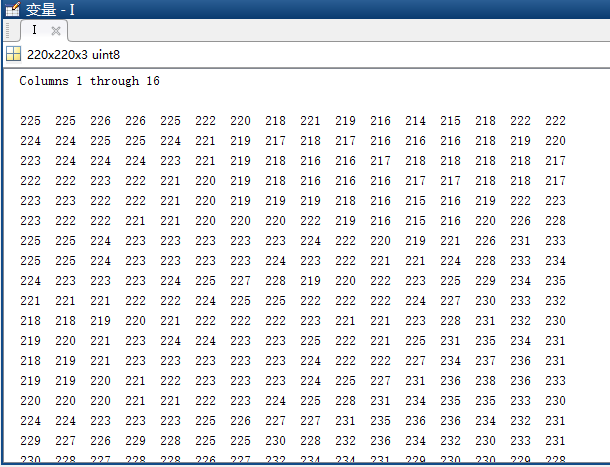然而计算机对于雾这种东西的理解，才是本文的精髓，这里要引出一个概念：直方图。直方图是指对图片进行颜色分类后，反映不同颜色出现次数的统计情况（也有人说是统计灰度的，这里说统计色彩为了更好理解），如肉眼所见，雾霾占据了整张图片的大部分面积，把整体的颜色拖向偏白的颜色，这使得该图的直方图变得更为集中于一个区域——白色

如此说来根据计算机对雾霾的理解，去除雾霾的原理，就是让图像的直方图从集中于白色的状态，转化成均匀的分布的状态，这样，图像才会回到原来丰富的色彩，雾霾占据的地方就会变得更加清晰了，然而matlab已然已经准备好了相关的函数histeq（参数是一个uint8的数组）。（终于可以说代码的事了。。。。)核心代码如下：function In = removeFogByGlobalHisteq(I)
%removeFogByGlobalHisteq 通过全局平衡直方图对图像去雾处理
%  I 输入图像
%拆分三原色
R=I(:,:,1);
G=I(:,:,2);
B=I(:,:,3);
%取直方图平衡
M=histeq(R);
L=histeq(G);
N=histeq(B);
%融合直方图
In=cat(3,M,L,N);
%上面的代码就是去雾用的
%-----------------------
%下面的代码都是用于显示的
figure;
subplot(2,2,1);imshow(I);title('原图' ,'FontWeight','Bold');
subplot(2,2,2);imshow(In);title('处理后的图' ,'FontWeight','Bold');
Q=rgb2gray(I);%获取该图直方图
W=rgb2gray(In);%获取该图直方图

subplot(2,2,3);imhist(Q,64);title('原图直方图' ,'FontWeight','Bold');
subplot(2,2,4);imhist(W,64);title('处理后直方图' ,'FontWeight','Bold');
end

下面是效果图：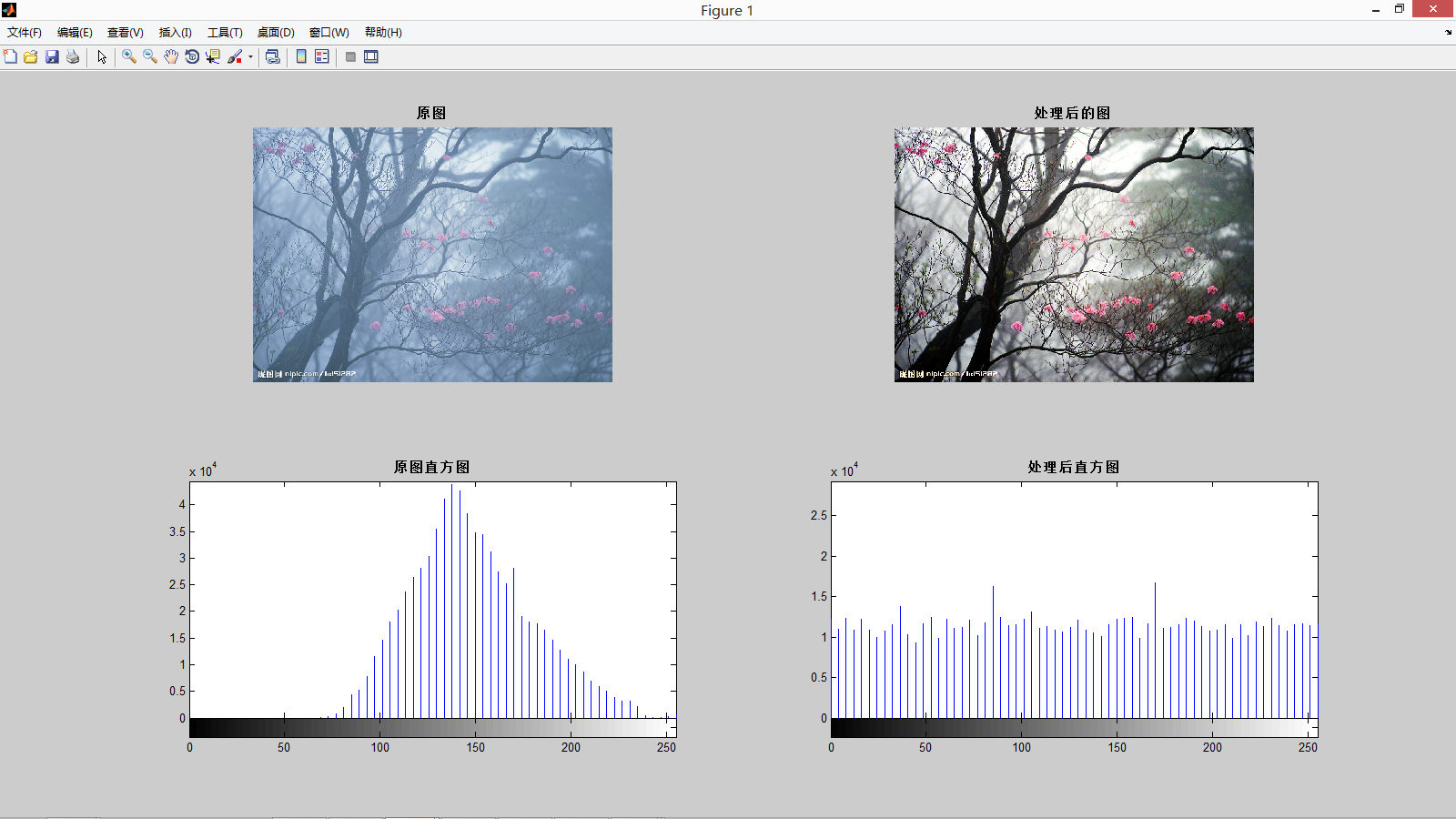（处理后的直方图明显平缓了很多。。）看到这一幕，是否很惊讶？！学会这招，强力对抗雾霾，闲来给妹子秀一发，形象瞬间从程序猿、修电脑的

一跃成为软件工程师和亲爱的有没有？千万不用谢我，应该的应该的。。。。（那啥，我的银行账号写这了哈：xxxxxxxxxxx）


展开全文图片 程序员 matlab 图像处理
• 1.1 MATLAB图像处理基本操作 本文中对于大多数的操作，是对数字图像处理领域中最为著名的“lena”图片进行操作的。原图如下（Figure 1）：  Figure 1 首先，在MATLAB中显示这幅图片： I=imread('lena.jpg')...
1.1  MATLAB图像处理基本操作

本文中对于大多数的操作，是对数字图像处理领域中最为著名的“lena”图片进行操作的。原图如下（Figure 1）：Figure 1

首先，在MATLAB中显示这幅图片：

I=imread('lena.jpg');
imfinfo('lena.jpg')
imshow(I);Figure 2

从Figure 2中可以看出，该图片格式为png，长宽均为512px，颜色类型为真彩色。

处理后的图片还需要进行保存：

imwrite(I,'lenaSave.jpg');

1.2  图像数字化

为了完成这样一个步骤，MATLAB中提供了相应的函数：

I1=rgb2gray(I);
imshow(I1);

I1以512*512*1的矩阵形式进行存储。效果如下图（Figure 3）：Figure 3

1.3    直方图

为了显示图像灰度的分布情况，还需要绘制灰度直方图。可以使用如下代码：

figure,imhist(I1);%显示图片灰度值的统计结果直方图

效果如下（Figure 4）：Figure 4

1.4   图像噪声的添加

为了完成多种图像处理的操作和试验，还可以对图片添加噪声。所用函数为imnoise (I, type)，该函数中的type可以为5种噪声参数，分别为：'gaussian'(高斯白噪声)，'localvar'(与图象灰度值有关的零均值高斯白噪声)，'poisson'(泊松噪声)，'salt & pepper'(椒盐噪声)和'speckle'(斑点噪声)。

以下为高斯噪声（Figure 5）和椒盐噪声（Figure 6）的代码和效果：

I2=imnoise(I1,'gaussian');
figure,imshow(I2);Figure 5

I3=imnoise(I1,'salt & pepper');
figure,imshow(I3);Figure 6


展开全文• GUI，即图形用户接口，是MATLAB的可视化操作功能，本文编写一个简单GUI进行图像处理。1、新建GUI文件，点击file-&gt;new-&gt;GUI如下图所示点击GUI后出现如下图界面，默认为Blank GUI (Default)选项，点击OK...
GUI，即图形用户接口，是MATLAB的可视化操作功能，本文编写一个简单GUI进行图像处理。1、新建GUI文件，点击file->new->GUI如下图所示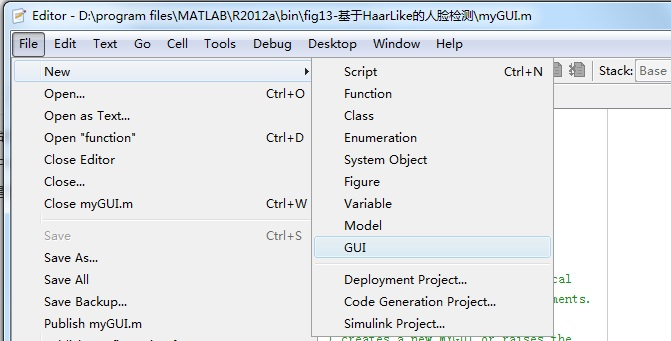点击GUI后出现如下图界面，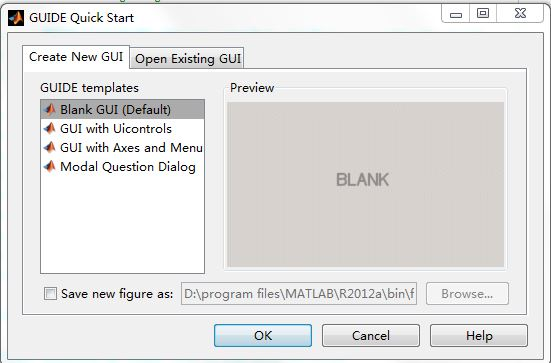默认为Blank  GUI (Default)选项，点击OK即可，出现如下图所示文件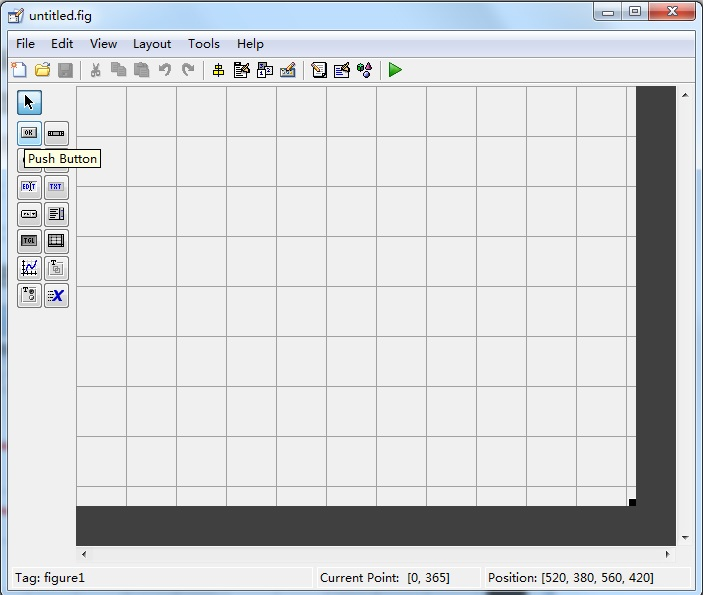将鼠标放到OK的位置会显示Push Button，为按钮，点击后在格子区域放置按钮，双击按钮，在string条目设置字符串“选择图片”，如下图所示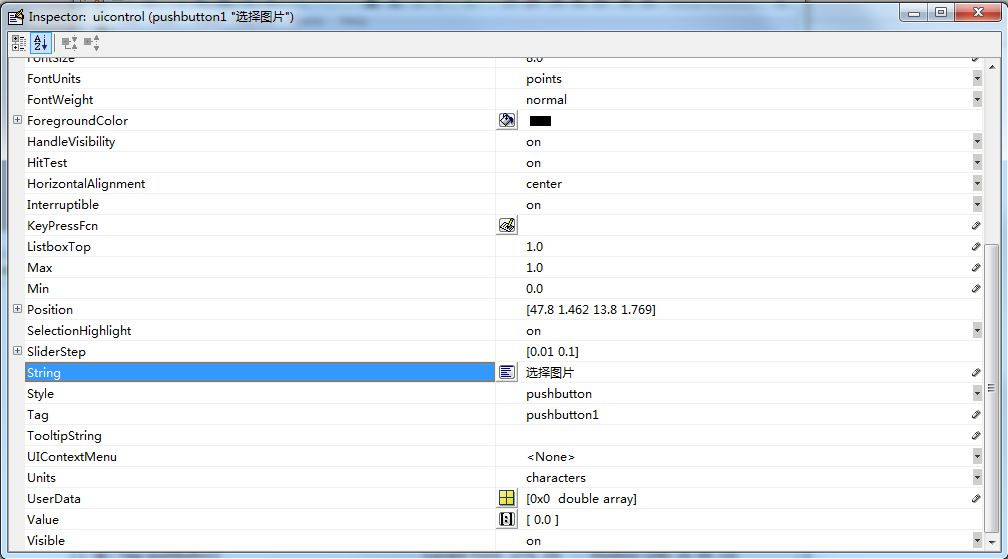然后放置可编辑文本edit和坐标系统axes，如下图所示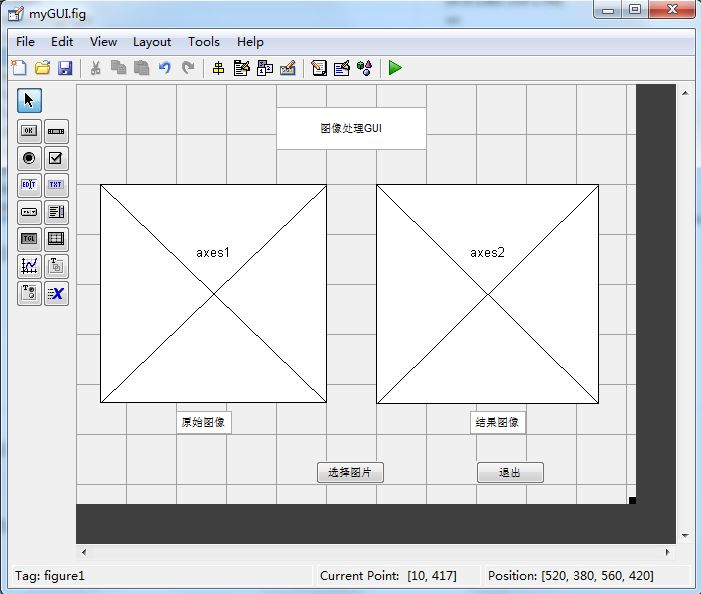保存命名为myGUI。2、编辑按钮响应事件，双击按钮，在callback条目行点击红框处，如图所示，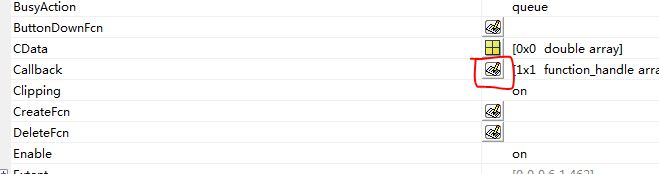在随后出现的myGUI.m文件中找到函数function pushbutton1_Callback，编写如下所示代码axis off  %%关闭坐标轴显示
[filename pathname] =uigetfile({'*.jpg';'*.bmp';'*.*'},'打开图片');

str=[pathname filename];
%%打开图像
%%打开axes1的句柄 进行axes1的操作
axes(handles.axes1);
%%在axes1中显示 图像
imshow(im);

%图像处理部分
I=im2bw(im);
BW1=edge(I,'canny'); %用canny算子进行边缘检测
path1='D:\program files\MATLAB\R2012a\bin\fig13-基于HaarLike的人脸检测\result\';
name='lena.jpg';
imwrite(BW1,[path1 name]);

str1=[path1 name];
axes(handles.axes2);
imshow(im1);  点击运行，出现如下图所示界面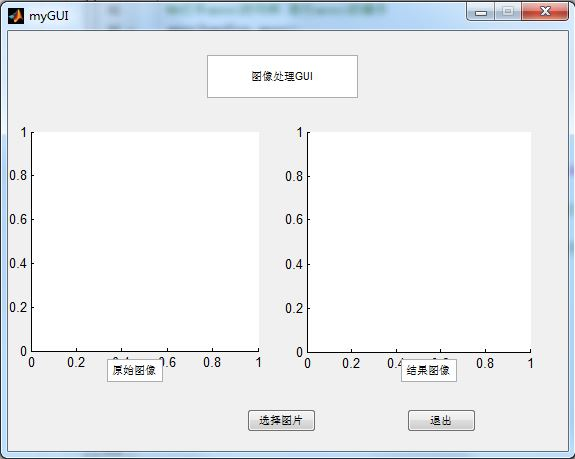在上图中点击“选择图片”，选择你想处理的图，然后在“结果图像”坐标系内显示图像处理的结果，如下图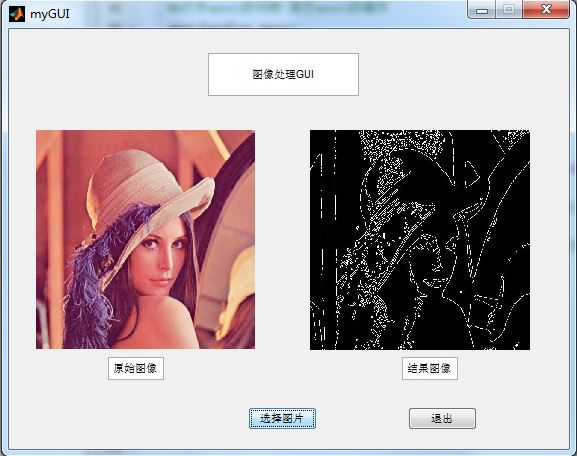展开全文MATLAB 图像处理 GUI
• 写在前头：说到数字图像处理，不得不提起MATLAB。这是一款非常方便的仿真软件，绝大多数的图像处理可以用MATLAB完成。 　有人问，处理图片，用PS岂不是更好。两者各有优点，如果需要将1００００幅图片转换成灰度...
写在前头：说到数字图像处理，不得不提起MATLAB。这是一款非常方便的仿真软件，绝大多数的图像处理可以用MATLAB完成。
有人问，处理图片，用PS岂不是更好。两者各有优点，如果需要将1００００幅图片转换成灰度图像并保存呢？MATLAB只需要一段很短的程序运行几秒就可以完成这个工作。
本文基于MatlabR2012a，将由浅入深写下去。

MATLAB中图像的基本操作

1、读取、显示图片

imread：
A = imread(filename, fmt)

A是结构体名，用来存储读入的图像数据。filename是读取的文件名，文件名要用”括起来。fmt是读取文件的类型如：jpg、png等等，这个参数可以不输入，由MATLAB自动判断。
显示函数imshow()原型：

    imshow：
imshow(I)

I为读取后保存在MATLAB中的结构体名。
程序实例：

A=imread('1.jpg');％读取名为１．ｊｐｇ的图片
imshow(A)％显示图片２、将灰度图片变成负片

对图像进行操作，实际上是将图像看成许多个像素点，对每个像素点进行操作。在计算机系统中，灰度图片被看成是许多个由值在［０～２５５］之间的像素点组成的图像，２５５表示白色，０表示黑色，黑白之间存在２５６个灰度级。负片是指将原灰度图白色的地方变成黑色，黑色的地方变成白色。也就是将０变成２５５，２５５变成０。ＭＡＬＴＡＢ的ｉｍａｄｊｕｓｔ（）函数提供了该功能。其函数原型：

imadjust：
J = imadjust(I,[low_in; high_in],[low_out; high_out])

其中，Ｉ为原灰度图像，ｌｏｗ＿ｉｎ，ｈｉｇｈ＿ｉｎ为输入图像的低和高灰度级，设置为［０，１］表示从０～２５５的归一化，ｌｏｗ＿ｏｕｔ，ｈｉｇｈ＿ｏｕｔ为输出图像的低高灰度级。
若是想将图片转换为负片，那么将[low_in; high_in]设置为［０，１］，将[low_out; high_out]设置为［１，０］。即原来输入为０的地方变成１输出，输入为１的地方变成０输出。
实例：

　A=imread('1.jpg');
imshow(A)％显示原图
figure,imshow(A1)％显示负片３、彩色图片转换成灰度图片

我们在计算机中看到的大多数彩色图片是基于ＲＢＧ三通道的图片，红绿蓝三种颜色，每一种取值均为［０～２５５］。通过２５５＊２５５＊２５５，可以构成庞大的颜色群。而灰度图像只有２５６个灰度级。通过以下公式可以将ＲＧＢ转换成灰度
ＧＲＡＹ＝0.2989  R + 0.5870 * G + 0.1140 * B*
ＭＡＴＬＡＢ中提供的函数ｒｇｂ２ｇｒａｙ为我们提供了将彩色图片转换成灰度图片的功能。函数原型：

I = rgb2gray(RGB)

实例：

 A=imread('001.png');％原彩色图片
imshow(A)
A_gray=rgb2gray(A);％转换成灰度图片
figure,imshow(A_gray)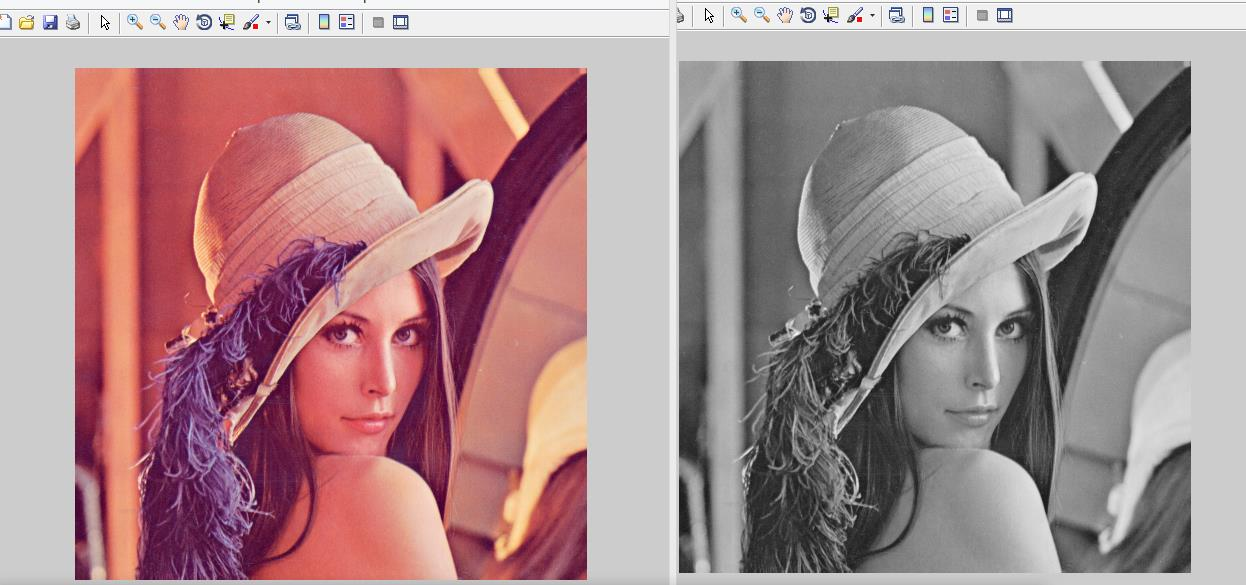展开全文图像处理 matlab 图片
• 1.基本函数: iread('文件路径'); idisp(); 2.一元操作： imd=idouble(im);... //将彩色图像转变成灰度图像。 ihist（）；//显示每一个像素值出现的次数 im=igamma(im,1/0.45); //伽马编码，一种非线性一...
• 一、imfinfo函数——查看图像文件信息，注意参数是文件路径和文件名，不是图像对应的矩阵。 &gt;&gt; imfinfo('C:\Users\zhulf\Desktop\1.jpg') ans =   Filename: 'C:\Users\zhulf\Desktop\1.jpg'  ...matlab 图像处理
• 对于图像的平移，MATLAB中是可以利用膨胀函数平移图像。 代码： I = imread('a1.jpg'); se = translate(strel(1), [50 140]);%将一个平面结构化元素分别向下和向右移动30个位置 J = imdilate(I,se);%利用膨胀函数平...MATLAB 数字图像处理
• 本书分为4部分，共29章，详细讲解了MATLAB的结构和功能，以及MATLAB图像处理工具箱。针对制作完整的图像处理软件的需要，本书还讲解了MATLAB界面编程。 本书第1部分“基础篇”，包括第1～第10章，对MATLAB安装试验...MATLAB图像
• 本书主要介绍MATLAB语言在图像处理技术方面的应用，重点介绍了各种图像处理方法的理论和实际应用，书中给出了大量MATLAB实例和图像处理效果，使渎职能够更好的理解和掌握使用MATLAB图像处理工具箱函数进行图像处理的...
• 平滑滤波——matlab图像处理 平滑滤波的目的是消除或尽量减少噪声，改善图像的质量。假设加性噪声是随机独立分布，这样利用图像像素领域的平均或加权平均即可有效地抑制噪声干扰。从信号分析的观点来看，图像平滑...平滑滤波 Matlab
• 第三章：MATLAB图像处理基础 第一章：MATLAB基础知识 1.1 MATLAB概述与桌面操作 1、matlab将数值分析、矩阵计算、科学数据可视化以及非线性动态系统的建模和仿真等诸多强大功能集成在一个易于使用的视窗环境中...
•图像处理 matlab
• MATLAB具有编程高效、易学易用的特点，是目前工程上流行最...它提供了图像处理和图像捕获工具箱，与MATLAB的数据分析和可视化环境集成在一起，可使专业人士从繁杂的编程中解脱出来，而集中在问题的分析与算法设计上。MATLAB 图像处理
• 涵盖了Matlab图像处理工具箱的几乎所以的M函数，他们扩张了Matlab解决图像处理问题的能力。相信这是对有志于数字图像处理的各路英雄是个好帮手！
• 利用中值滤波的方法对图像进行平滑处理，函数如下 fig_med = medfilt2(fig,[m,n]) % 对矩阵[m,n]进行中值滤波 类间方差阈值算法 利用类间方差阈值算法对滤波处理图像进行分割处理，获取分割图像； 子函数如下所...matlab 图像处理 图像分割
...